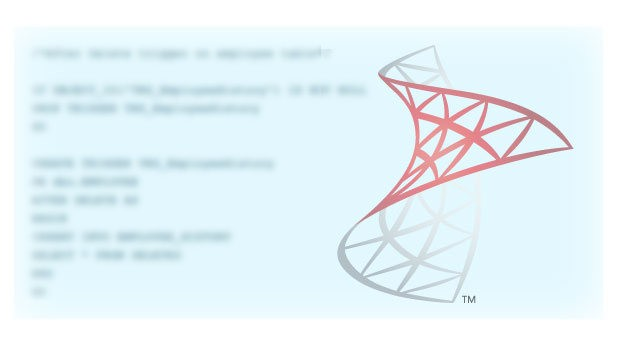# How To Use LEN and DATALENGTH Function In SQL Server

Posted March 4, 2019 by Vishwanath Dalvi in Database, SQL ServerCounting the number of characters or size of a string in SQL Server is possible with LEN and DATALENGTH function. Both these functions are used interchangeably. While we need to be careful while using these functions in production code. Basically, both the function sounds similar although each has a distinct purpose.

Unexpectedly, I have seen a production code where a developer used DATALENGTH function instead of LEN. In most cases, the DATALENGTH function worked without any hassle. Particularly someday, the code started giving wrong output. After analysis, we found that DATALENGTH on NVARCHAR data type returns different output compared to the VARCHAR type column.

In this tech-recipes post, we will learn LEN and DATALENGTH function along with their differences.

### LEN Function – Points to Ponder

LEN function returns the number of characters – excluding trailing spaces in a string.

1LEN function ignores the trailing spaces while counting the number of characters in a string.

2LEN function considers the leading spaces while counting the number of characters.

3On an empty string, the function returns 0 and NULL if the input string is NULL.

### DATALENGTH Function – Points to Ponder

DATALENGTH function returns the number of bytes used by expression of any data type. Importantly, the NVARCHAR data type takes 2 bytes for a single character and VARCHAR data type takes 1 byte only.

1DATALENGTH function counts the number of bytes in a string.

2Function considers the leading and trailing spaces while counting the number of bytes.

3The function returns BIGINT number if input data types include VARCHAR(MAX), NVARCHAR(MAX) else INT.

4For NULL input, it returns NULL as output and for EMPTY string returns 0.

### Examples

Let’s drive through with few examples using LEN and DATALENGTH function together to understand their working and difference.

1

### LEN – Working On String Expressions

In this example, we can learn that the LEN function ignores trailing spaces. However, it does count the leading spaces in a string.

```SELECT LEN('Tech Recipes')     as Len1  -- 12
,LEN('Tech Recipes    ') as Len2  -- 12 (Ignores trailing spaces)
,LEN('  Tech-Recipes')   as Len3; -- 14 (Considers leading spaces)
```

2.

### LEN – Considering Trailing Spaces

By default, LEN function doesn’t consider trailing spaces but in case we need to consider it. Using the following code we can achieve desired results – it considers if the input string contains maximum characters.

```DECLARE @String as VARCHAR(200) = 'Tech Recipes   ';

SELECT LEN(CAST(@String as VARCHAR(MAX)) + 'a') - 1 as Len1
,LEN(REPLACE(@String,' ','@')) as Len2 -- Most reliable method
,DATALENGTH(@String) as Len3;	-- Only useful on VARCHAR - Careful on NVARCHAR
```

### Difference – LEN vs DATALENGTH

Both these function act differently in various use cases. We will consider the number of characters, leading and trailing spaces. Moreover, different data types.

1.

In this example, we will demonstrate how LEN and DATALENGTH work differently on leading and trailing spaces. Note that LEN function ignores trailing spaces but considers leading spaces. Whereas DATALENGTH considers both leading and trailing spaces.

```SELECT LEN('Tech  ') as Len1, --Ignores trailing spaces
DATALENGTH('Tech  ') as Len2; --Includes trailing spaces;

SELECT LEN('  Tech') as Len1, --Includes leading spaces
DATALENGTH('  Tech') as Len2;--Includes leading spaces ;

SELECT LEN('  Tech  ') as Len1, --Includes leading but ignores trailing spaces
DATALENGTH('  Tech  ') as Len2; --Includes leading and trailing spaces
```

2.

### 2. Data Types

In brief, we are aware that VARCHAR data types take 1 byte to store a single character whereas NVARCHAR data type takes 2 bytes. Thus in this scenario, LEN and DATALENGTH function provides different output. LEN function counts the number of characters, and DATALENGTH function counts the number of bytes. In the case of NVARCHAR data type DATALENGTH function will return 2 x Number of characters.

### VARCHAR Data Type

Varchar data types take 1 byte per character thus DATALENGTH function returns 12. Moreover, the LEN function counts the number of characters as 12.

```--Varchar Type
DECLARE @VarcharString as VARCHAR(20) = 'Tech-Recipes';

SELECT  LEN(@VarcharString) as Len --12
,DATALENGTH(@VarcharString) as DataLen; --12 (each character 1 byte)
```

### NVARCHAR Data Type

In below example, we can briefly understand the difference. NVARCHAR data type takes 2 bytes to store single character thus for 12 characters we see 24 as output using DATALENGTH function.

```--Nvarchar Type
DECLARE @NvarcharString as NVARCHAR(20) = 'Tech-Recipes';

SELECT  LEN(@NvarcharString)
,DATALENGTH(@NvarcharString);--24 as each character takes 2 byte (12*2 byte=24 bytes)
GO
```

### Char Data Type

In below example, following the functioning of CHAR data type where extra characters are padded to string thus it returns 20 using DATALENGTH function and 4 using LEN function.

```--Char Type
DECLARE @CharString as CHAR(20) = 'test';

SELECT LEN(@CharString) as Len,
DATALENGTH(@CharString) as DataLen; --20 as CHAR type occupies entire storage
```

### Summary

As a result we have leared to use LEN and DATALENGTH function in SQL Server. Using both these function we can count the number of characters and size of string. If you like this article you may read through Tech-recipes database archives.